On-line GuidesAll GuideseBook StoreiOS / AndroidLinux for BeginnersOffice ProductivityLinux InstallationLinux SecurityLinux UtilitiesLinux VirtualizationLinux KernelSystem/Network AdminProgrammingScripting LanguagesDevelopment ToolsWeb DevelopmentGUI Toolkits/DesktopDatabasesMail SystemsopenSolarisEclipse DocumentationTechotopia.comVirtuatopia.comHow To GuidesVirtualizationGeneral System AdminLinux SecurityLinux FilesystemsWeb ServersGraphics & DesktopPC HardwareWindowsProblem SolutionsPrivacy Policy## Object Collaboration

Object-oriented programming helps us by encapsulating data and processing into a tidy class definition. This encapsulation assures us that our data is processed correctly. It also helps us understand what a program does by allowing us to ignore the details of an object's implementation.

When we combine multiple objects into a collaboration, we exploit the power of ecapsulation. We'll look at a simple example of creating a composite object, which has a number of detailed objects inside it.

Defining Collaboration. Defining a collaboration means that we are creating a class which depends on one or more other classes. Here's a new class, `Dice`, which uses instances of our `Die` class. We can now work with a `Dice` collection, and not worry about the details of the individual `Die` objects.

Example 21.4. dice.py - part 1

```#!/usr/bin/env python
"""Define a Die, and Dice and simulate a dozen rolls."""

class Die( object ):

See the definition in Example 21.1, “die.py”.

class Dice( object ):
"""Simulate a pair of dice."""
def __init__( self ):
"Create the two Die objects."
self.myDice = ( Die(), Die() )
def roll( self ):
"Return a random roll of the dice."
for d in self.myDice:
d.roll()
def getTotal( self ):
"Return the total of two dice."
t= 0
for d in self.myDice:
t += d.getValue()
return t
def getTuple( self ):
"Return a tuple of the dice values."
return tuple( [d.getValue() for d in self.myDice] )
def hardways( self ):
"Return True if this is a hardways roll."
return self.myDice.getValue() == self.myDice.getValue()
```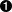This is the definition of a single `Die`, from the `die.py` example. We didn't repeat it here to save some space in the example.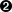This class, `Dice`, defines a pair of `Die` instances.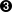The `__init__` method creates an instance variable, `myDice`, which has a tuple of two instances of the `Die` class.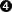The `roll` method changes the overall state of a given `Dice` object by changing the two individual `Die` objects it contains. This manipulator uses a for loop to assign each of the internal `Die` objects to `d`. In the loop it calls the `roll` method of the `Die` object, `d`. This technique is called delegation: a `Dice` object delegates the work to two individual `Die` objects. We don't know, or care, how each `Die` computes it's next value.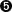The `getTotal` method computes a sum of all of the `Die` objects. It uses a for loop to assign each of the internal `Die` objects to `d`. It then uses the `getValue` method of `d`. This is the official interface method; by using it, we can remain blissfully unaware of how `Die` saves it's state.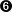The `getTuple` method returns the values of each `Die` object. It uses a list comprehension to create a `list` of the `value` instance variables of each `Die` object. The built-in function `tuple` converts the `list` into an immutable `tuple`.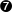The `harways` method examines the value of each `Die` objec to see if they are the same. If they are, the total was made "the hard way."

The `getTotal` and `getTuple` methods return basic attribute information about the state of the object. These kinds of methods are often called getters because their names start with “get”.

Collaborating Objects. The following function exercises an instance this class to roll a `Dice` object a dozen times and print the results.

```def test2():
x= Dice()
for i in range(12):
x.roll()
print x.getTotal(), x.getTuple()
```

This function creates an instance of `Dice`, called `x`. It then enters a loop to perform a suite of statements 12 times. The suite of statements first manipulates the `Dice` object using its `roll` method. Then it accesses the `Dice` object using `getTotal` and `getTuple` method.

Here's another function which uses a `Dice` object. This function rolls the dice 1000 times, and counts the number of hardways rolls as compared with the number of other rolls. The fraction of rolls which are hardways is ideally 1/6, 16.6%.

```def test3():
x= Dice()
hard= 0
soft= 0
for i in range(1000):
x.roll()
if x.hardways(): hard += 1
else: soft += 1
print hard/1000., soft/1000.
```

Independence. One point of object collaboration is to allow us to modify one class definition without breaking the entire program. As long as we make changes to Die that don't change the interface that Die uses, we can alter the implementation of Die all we want. Similarly, we can change the implementation of Dice, as long as the basic set of methods are still present, we are free to provide any alternative implementation we choose.

We can, for example, rework the definition of `Die` confident that we won't disturb `Dice` or the functions that use `Dice` (`test2` and `test3`). Let's change the way it represents the value rolled on the die. Here's an alternate implemetation of Die. In this case, the private instance variable, `value`, will have a value in the range `0<=value<=5`. When getValue adds 1, the value is in the usual range for a single die, 1≤ n ≤6.

```class Die(object):
"""Simulate a 6-sided die."""
def __init__( self ):
self.roll()
def roll( self ):
self.value= random.randint(0,5)
retuen self.value
def getValue( self ):
return 1+self.value
```

Since this version of `Die` has the same interface as other versions of `Die` in this chapter, it is isomorphic to them. There could be performance differences, depending on the performance of `randint` and `randrange` functions. Since `randint` has a slightly simpler definition, it may process more quickly.

Similarly, we can replace `Die` with the following alternative. Depending on the performance of `choice`, this may be faster or slower than other versions of `Die`.

```class Die(object):
"""Simulate a 6-sided die."""
def __init__( self ):
self.domain= range(1,7)
def roll( self ):
self.value= random.choice(self.domain)
return self.value
def getValue( self ):
return self.value
```Published under the terms of the Open Publication License Design by Interspire